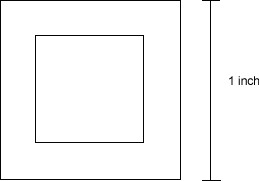# GRE Question 1

Today's question of the day is from the math category. Click on an answer choice and then click on 'submit' to see if you got it right!

In the figure below, if the area of the smaller square region is 2/3 the area of the larger square region, then the diagonal of the larger square is how many inches longer than the diagonal of the smaller square?